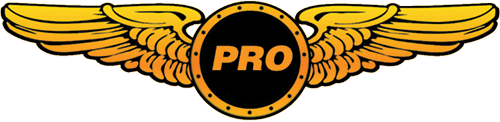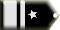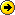# Default Boeing 747Chris102 Chief Captain

Could someone e-mail me the CFG file for the b747_400? If so, let me know so I can give you my e-mail address.tomthetank Chief Captain

Copy and paste[fltsim.0]
title=Boeing 747-400
sim=Boeing747-400
model=
panel=
sound=
texture=
kb_checklists=Boeing747-400_check
kb_reference=Boeing747-400_ref
atc_id=N747
atc_airline=Global Freightways
atc_flight_number=1123
atc_heavy=1
atc_parking_types=CARGO
ui_manufacturer=Boeing
ui_type="747-400"
ui_variation="Global Freightways"
description="More than 30 years ago, the 747 made its first trip from New York to London. Since then, it's become the standard by which other large passenger jets are judged. Its size, range, speed and capacity were then, and are now, the best in its class."

[fltsim.1]
title=Boeing 747-400 Paint1
sim=Boeing747-400
model=
panel=
sound=
texture=1
kb_checklists=Boeing747-400_check
kb_reference=Boeing747-400_ref
atc_id=N747K
atc_airline=Landmark
atc_flight_number=1123
atc_heavy=1
ui_manufacturer=Boeing
ui_type="747-400"
ui_variation="Landmark Airlines"
description="More than 30 years ago, the 747 made its first trip from New York to London. Since then, it's become the standard by which other large passenger jets are judged. Its size, range, speed and capacity were then, and are now, the best in its class."

[fltsim.2]
title=Boeing 747-400 Paint2
sim=Boeing747-400
model=
panel=
sound=
texture=2
kb_checklists=Boeing747-400_check
kb_reference=Boeing747-400_ref
atc_id=N747I
atc_airline=World Travel
atc_flight_number=1123
atc_heavy=1
ui_manufacturer=Boeing
ui_type="747-400"
ui_variation="World Travel Airlines"
description="More than 30 years ago, the 747 made its first trip from New York to London. Since then, it's become the standard by which other large passenger jets are judged. Its size, range, speed and capacity were then, and are now, the best in its class."

[fltsim.3]
title=Boeing 747-400 Paint3
sim=Boeing747-400
model=
panel=
sound=
texture=3
kb_checklists=Boeing747-400_check
kb_reference=Boeing747-400_ref
atc_id=N747P
atc_airline=Soar
atc_flight_number=1123
atc_heavy=1
ui_manufacturer=Boeing
ui_type="747-400"
ui_variation="Soar Airlines"
description="More than 30 years ago, the 747 made its first trip from New York to London. Since then, it's become the standard by which other large passenger jets are judged. Its size, range, speed and capacity were then, and are now, the best in its class."

[General]
atc_type=BOEING
atc_model=B744
editable=0
performance="Cruise Speed\n0.85 Mach 565 mph 910 km\/h\n\nEngine options\nPratt & Whitney PW4062\nRolls-Royce RB211-524H\nGeneral Electric CF6-80C2B5F\n\nMaximum Range\n7,325 nm 13,570 km\n\nMaximum Certified Operating Altitude 45,100 ft 13,747 m\n\nFuel Capacity\n57,285 gal 216,840 L\n\nBasic Empty Weight\n394,088 lb 178,755 kg\n\nMax Gross Weight 875,000 lb 396,893 kg\n\nLength\n231 ft, 10 in 70.6 m\n\nWingspan\n211 ft, 5 in 64.4 m\n\nHeight\n63 ft, 8 in 19.4 m\n\nSeating Typical 3-class configuration - 416\nTypical 2-class configuration - 524"

[pitot_static]
vertical_speed_time_constant = 1 //Increasing this value will cause a more instantaneous reaction in the VSI

[WEIGHT_AND_BALANCE]
max_gross_weight = 875000 // (pounds)
empty_weight = 394088 // (pounds)

reference_datum_position = 83.5, 0, 0 // (feet) distance from FlightSim Reference position: (1/4 chord, centerline, waterline)
empty_weight_CG_position = -90.5, 0, 0 // (feet) longitudinal, lateral, vertical distance from specified datum

max_number_of_stations = 50

station_load.0 = "170.0, -19.0, -2.0, 8.0, Pilot" //Weight (lbs), longitudinal, lateral, vertical positions from datum (feet)
station_load.1 = "170.0, -19.0, 2.0, 8.0, Co-Pilot" //Weight (lbs), longitudinal, lateral, vertical positions from datum (feet)
station_load.2 = "1700.0, -83.5, 0.0, 0.0, Crew" //Weight (lbs), longitudinal, lateral, vertical positions from datum (feet)
station_load.3 = "5440.0, -27.0, 0.0, 0.0, First Class" //Weight (lbs), longitudinal, lateral, vertical positions from datum (feet)
station_load.4 = "5780.0, -65.0, 0.0, 0.0, Business Class" //Weight (lbs), longitudinal, lateral, vertical positions from datum (feet)
station_load.5 = "7140.0, -50.0, 0.0, 8.0, Upper Deck" //Weight (lbs), longitudinal, lateral, vertical positions from datum (feet)
station_load.6 = "10710.0, -91.0, 0.0, 0.0, Coach 1-7" //Weight (lbs), longitudinal, lateral, vertical positions from datum (feet)
station_load.7 = "18020.0, -119.0, 0.0, 0.0, Coach 8-18" //Weight (lbs), longitudinal, lateral, vertical positions from datum (feet)
station_load.8 = "23800.0, -160.0, 0.0, 0.0, Coach 19-34" //Weight (lbs), longitudinal, lateral, vertical positions from datum (feet)
station_load.9 = "18000.0, -52.0, 0.0, -6.0, Forward Baggage" //Weight (lbs), longitudinal, lateral, vertical positions from datum (feet)
station_load.10 = "6000.0, -123.0, 0.0, -6.0, Aft Baggage" //Weight (lbs), longitudinal, lateral, vertical positions from datum (feet)

;Moments of Inertia
empty_weight_roll_MOI = 13352310
empty_weight_pitch_MOI = 24223159
empty_weight_yaw_MOI = 39531785
empty_weight_coupled_MOI = 0

[fuel]
LeftMain = -96.0, -28.0, -4.0, 12546.0, 0.0 //Longitudinal (feet), Lateral (feet), Vertical (feet), Usable(gallons), Unusable (gallons)
RightMain = -96.0, 28.0, -4.0, 12546.0, 0.0 //Longitudinal (feet), Lateral (feet), Vertical (feet), Usable(gallons), Unusable (gallons)
LeftAux = -112.0, -36.5, -3.0, 4482.0, 0.0 //Longitudinal (feet), Lateral (feet), Vertical (feet), Usable(gallons), Unusable (gallons)
RightAux = -112.0, 36.5, -3.0, 4482.0, 0.0 //Longitudinal (feet), Lateral (feet), Vertical (feet), Usable(gallons), Unusable (gallons)
LeftTip = -130.0, -73.0, 0.0, 1322.0, 0.0 //Longitudinal (feet), Lateral (feet), Vertical (feet), Usable(gallons), Unusable (gallons)
RightTip = -130.0, 73.0, 0.0, 1322.0, 0.0 //Longitudinal (feet), Lateral (feet), Vertical (feet), Usable(gallons), Unusable (gallons)
Center1 = -83.5, 0.0, -7.0, 17164.0, 0.0 //Longitudinal (feet), Lateral (feet), Vertical (feet), Usable(gallons), Unusable (gallons)
Center2 = -193.5, 0.0, 6.0, 3300.0, 0.0 //Longitudinal (feet), Lateral (feet), Vertical (feet), Usable(gallons), Unusable (gallons)

fuel_type = 2 //Fuel type: 1 = Avgas, 2 = JetA
number_of_tank_selectors = 1
electric_pump=0

[airplane_geometry]
wing_area = 5825.0 //Square feet
wing_span = 211.4 //Feet
wing_root_chord = 48.8 //Feet
wing_dihedral = 7.0 //Degrees
wing_incidence = 2.0 //Degrees
wing_twist = -1.0 //Degrees
oswald_efficiency_factor= 0.68 //Measure of lift effeciency of wing
wing_winglets_flag = 1 //Are winglets available?
wing_sweep = 37.5 //Degrees, wing leading edge
wing_pos_apex_lon = -58.2 //Feet, longitudinal distance from reference point, negative going aft
wing_pos_apex_vert = 0 //Feet, vertical distance from reference point, positive going up
htail_area = 1470 //Square feet
htail_span = 72.8 //Feet
htail_pos_lon = -210.0 //Feet, longitudinal distance from reference point, negative going aft
htail_pos_vert = 0.0 //Feet, vertical distance from reference point, positive going up
htail_incidence = 0.0 //Degrees
htail_sweep = 37.5 //Degrees, horizontal tail leading edge
vtail_area = 1060 //Square feet
vtail_span = 37.1 //Feet, tip to body
vtail_sweep = 45.0 //Degrees, vertical tail leading edge
vtail_pos_lon = -198.5 //Feet, longitudinal distance from reference point, negative going aft
vtail_pos_vert = 26.1 //Feet, vertical distance from reference point, positive going up
elevator_area = 327 //Square feet
aileron_area = 225 //Square feet
rudder_area = 230 //Square feet
elevator_up_limit = 25 //Degrees
elevator_down_limit = 15 //Degrees
aileron_up_limit = 25 //Degrees
aileron_down_limit = 15 //Degrees
rudder_limit = 31.5 //Degrees
elevator_trim_limit = 20 //Degrees
spoiler_limit = 45 //Degrees
spoilerons_available = 1 //Spoilerons Available?
aileron_to_spoileron_gain = 3 //Aileron to spoileron gain
min_ailerons_for_spoilerons = 10 //Degrees
min_flaps_for_spoilerons = 0 //Minimum flap handle position when spoilerons activate

[Reference Speeds]
flaps_up_stall_speed = 140.0 //Knots True (KTAS)
full_flaps_stall_speed = 112.0 //Knots True (KTAS)
cruise_speed = 505.0 //Knots True (KTAS)
max_mach = 0.92
max_indicated_speed = 365.0 //Red line (KIAS)

[flaps.0]
type = 1 // 1 - tail, 2 - lead
span-outboard = 0.8 // 0.0 .. 1.0
extending-time = 25 // seconds
flaps-position.0 = 0 // degrees
flaps-position.1 = 1 // degrees
flaps-position.2 = 5 // degrees
flaps-position.3 = 10 // degrees
flaps-position.4 = 20 // degrees
flaps-position.5 = 25 // degrees
flaps-position.6 = 30 // degrees
damaging-speed = 200 // KIAS
blowout-speed = 250 // KIAS
lift_scalar = 0.7
drag_scalar = 0.9
pitch_scalar= 0.9
system_type = 1 //Hydraulic

[flaps.1]
type = 2 // 1 - tail, 2 - lead
span-outboard = 0.8 // 0.0 .. 1.0
extending-time = 2 // seconds
flaps-position.0 = 0 // degrees
flaps-position.1 = 1.0 // degrees
damaging-speed = 250 // KIAS
blowout-speed = 300 // KIAS
lift_scalar = 0.3
drag_scalar = 0.1
pitch_scalar= 0.1
system_type = 1 //Hydraulic

[flight_tuning]
cruise_lift_scalar = 1.0
parasite_drag_scalar = 1.0
induced_drag_scalar = 1.0
elevator_effectiveness = 1.0
aileron_effectiveness = 1.0
rudder_effectiveness = 1.0
pitch_stability = 1.0
roll_stability = 1.0
yaw_stability = 1.0
elevator_trim_effectiveness = 1.0
aileron_trim_effectiveness = 1.0
rudder_trim_effectiveness = 1.0

[GeneralEngineData]
engine_type = 1 //0=Piston, 1=Jet, 2=None, 3=Helo-Turbine, 4=Rocket, 5=Turboprop
Engine.0 = -107.5, -69.5, -6.9 //(feet) longitudinal, lateral, vertical distance from reference datum
Engine.1 = -76.0, -38.9, -10.4 //(feet) longitudinal, lateral, vertical distance from reference datum
Engine.2 = -76.0, 38.9, -10.4 //(feet) longitudinal, lateral, vertical distance from reference datum
Engine.3 = -107.5, 69.5, -6.9 //(feet) longitudinal, lateral, vertical distance from reference datum
fuel_flow_scalar = 1.0 //Scalar for fuel flow efficiency
min_throttle_limit = -0.50; //Minimum percent throttle. Generally negative for turbine reverser

[TurbineEngineData]
fuel_flow_gain = 0.002 //Gain on fuel flow
inlet_area = 60.0 //Square Feet, engine nacelle inlet area
rated_N2_rpm = 29920 //RPM, second stage compressor rated value
static_thrust = 56750 //Lbs, max rated static thrust at Sea Level
afterburner_available = 0 //Afterburner available?
reverser_available = 1 //Thrust reverser available?

[jet_engine]
thrust_scalar = 1.0

[autopilot]
autopilot_available = 1
flight_director_available = 1
default_vertical_speed = 1800.0
autothrottle_available = 1
autothrottle_arming_required = 1
autothrottle_max_rpm = 90
autothrottle_takeoff_ga = 1
pitch_takeoff_ga = 8;
max_pitch=10.0
max_pitch_acceleration=1.0
max_pitch_velocity_lo_alt=2.0
max_pitch_velocity_hi_alt=1.5
max_pitch_velocity_lo_alt_breakpoint=20000.0
max_pitch_velocity_hi_alt_breakpoint=28000.0
max_bank=25.0
max_bank_acceleration=1.8
max_bank_velocity=3.00
max_throttle_rate=0.10
nav_proportional_control=16.00
nav_integrator_control=0.17
nav_derivative_control=0.00
nav_integrator_boundary=2.50
nav_derivative_boundary=0.00
gs_proportional_control = 18.0
gs_integrator_control = 0.33
gs_derivative_control = 0.00
gs_integrator_boundary = 0.70
gs_derivative_boundary = 0.00
yaw_damper_gain = 1.0

[contact_points]

//0 Class <0=none,1=wheel, 2=scrape, 3=float>
//1 Longitudinal Position (feet)
//2 Lateral Position (feet)
//3 Vertical Position (feet)
//4 Impact Damage Threshold (Feet Per Minute)
//5 Brake Map (0=None, 1=Left, 2=Right)
//7 Steer Angle (degrees)
//8 Static Compression (feet) (0 if rigid)
//9 Max/Static Compression Ratio
//10 Damping Ratio (0=Undamped, 1=Critically Damped)
//11 Extension Time (seconds)
//12 Retraction Time (seconds)
//13 Sound Type
//14 Airspeed limit for retraction (KIAS)
//15 Airspeed that gear gets damage at (KIAS)

point.0 = 1, -25.0, 0.0, -17.5, 1000.0, 0, 2.0, 70.0, 0.5, 3.5, 0.900, 9.0, 8.0, 0, 220, 250
point.1 = 1, -114.0, -18.0, -21.3, 2000.0, 1, 2.0, 13.0, 3.0, 2.5, 0.900, 11.0, 9.0, 2, 220, 250
point.2 = 1, -114.0, 18.0, -21.3, 2000.0, 2, 2.0, 13.0, 3.0, 2.5, 0.900, 11.0, 9.0, 3, 220, 250
point.3 = 2, -152.6, -103.5, 3.0, 700.0, 0, 0.0, 0.0, 0.0, 0.0, 0.000, 0.0, 0.0, 5, 0, 0
point.4 = 2, -152.6, 103.5, 3.0, 700.0, 0, 0.0, 0.0, 0.0, 0.0, 0.000, 0.0, 0.0, 6, 0, 0
point.5 = 2, 3.0, 0.0, 0.0, 700.0, 0, 0.0, 0.0, 0.0, 0.0, 0.000, 0.0, 0.0, 9, 0, 0
point.6 = 2, -222.7, 0.0, 4.0, 700.0, 0, 0.0, 0.0, 0.0, 0.0, 0.000, 0.0, 0.0, 4, 0, 0

static_pitch = -1.5
static_cg_height = 18.6
gear_system_type=1 //Hydraulic

[gear_warning_system]
gear_warning_available = 1 //Normal
pct_throttle_limit = 0.1 //Percent throttle that activates warning
flap_limit_idle = 5.0 //Flap angle that activates warning at idle
flap_limit_power = 25.5 //Flap angle that activates warning at above idle

[brakes]
parking_brake = 1 //Parking brake available
toe_brakes_scale = 1.24 //Brake scalar

[hydraulic_system]
normal_pressure = 3000.0 //PSI
electric_pumps = 0 //no electric pumps
engine_map = 1,1,1,1 //pumps on Eng1,2,3,4

[Views]
VIEW_REAR_RIGHT_WINDOWS=32
VIEW-REAR_RIGHT_ZOOM=1.0
VIEW_REAR_RIGHT_EYE=-1.7, -0.3, -22
VIEW_REAR_RIGHT_DIR=-2.0, 0.0, 90.0

VIEW_REAR_WINDOWS=33

VIEW_REAR_LEFT_WINDOWS=34
VIEW_REAR_LEFT_ZOOM=1.0
VIEW_REAR_LEFT_EYE=1.7, -0.3, -22
VIEW_REAR_LEFT_DIR=-2.0, 0.0, 270.0

eyepoint = -19.05, -1.97, 9.7 ; Longitude, Latitude and Vertical position from Reference Point, (FEET)

[electrical]
autopilot = 0, 5 , 17.0
avionics_bus = 0, 5 , 17.0
avionics = 1, 5 , 17.0
gear_warning = 0, 2 , 17.0
fuel_pump = 0, 5 , 17.0
light_nav = 0, 5 , 17.0
light_beacon = 0, 5 , 17.0
light_landing = 0, 5 , 17.0
light_taxi = 0, 5 , 17.0
light_strobe = 0, 5 , 17.0
light_panel = 0, 5 , 17.0

// Radio Type = availiable, standby frequency, has glide slope
Audio.1 = 1
Com.1 = 1, 1
Com.2 = 1, 1
Nav.1 = 1, 1, 1
Nav.2 = 1, 1, 0
Transponder.1 = 1
Marker.1 = 1

[LIGHTS]
//Types: 1=beacon, 2=strobe, 3=navigation, 4=cockpit, 5=landing
light.0 = 3, -150.30, -102.56, 3.22, fx_navredh ,
light.1 = 3, -150.30, 102.56, 3.22, fx_navgreh ,
light.2 = 3, -223.85, 0.00, 8.85, fx_navwhih ,
light.3 = 2, -150.30, -102.56, 3.22, fx_strobeh ,
light.4 = 2, -150.30, 102.56, 3.22, fx_strobeh ,
light.5 = 2, -228.97, 0.00, 44.62, fx_strobeh ,
light.6 = 1, -45.05, 0.00, 14.65, fx_beaconh ,
light.7 = 1, -80.22, 0.00, -12.05, fx_beaconb ,
light.8 = 4, -16.24, 0.00, 9.19, fx_vclighth,

[direction_indicators]
//1 Type: 0=None, 1=Vacuum Gyro, 2=Electric Gyro, 3=Electro-Mag Slaved Compass, 4=Slaved to another
//2 Indicator number of slaving indicator if Type = 4
direction_indicator.0=3,0

[attitude_indicators]
//Type: 0=None, 1=Vacuum Gyro, 2=Electric Gyro
attitude_indicator.0 = 2
attitude_indicator.1 = 1

[turn_indicators]
//Type: 0=None, 1=Electric Gyro, 2= Vacuum Gyro
turn_indicator.0=0,0

[keyboard_response]
//Breakpoint speeds (knots) on the keyboard increments of control surfaces.
//Speed at which the incremenet is reduced to 1/2 and 1/8 respectively.
elevator = 150, 250
aileron = 150, 250
rudder = 150, 250

[EFFECTS]
wake=fx_wake
water=fx_spray
dirt=fx_tchdrt
concrete=fx_sparks
touchdown=fx_tchdwn, 1

[exits]
number_of_exits = 2
exit_rate.0 = 0.4 ;Percent per second
exit_rate.1 = 0.4 ;Percent per second

[forcefeedback]
gear_bump_nose_magnitude=3000 ; 0 - 10000
gear_bump_nose_direction=18000 ; 0 - 35999 degrees
gear_bump_nose_duration=250000 ; in microseconds
gear_bump_left_magnitude=2700 ; 0 - 10000
gear_bump_left_direction=35500 ; 0 - 35999 degrees
gear_bump_left_duration=250000 ; in microseconds
gear_bump_right_magnitude=2700 ; 0 - 10000
gear_bump_right_direction=00500 ; 0 - 35999 degrees
gear_bump_right_duration=250000 ; in microseconds
ground_bumps_magnitude1=1300 ; 0 - 10000
ground_bumps_angle1=08900 ; 0 - 35999 degrees
ground_bumps_intercept1=3.0
ground_bumps_slope1=0.20
ground_bumps_magnitude2=200 ; 0 - 10000
ground_bumps_angle2=09100 ; 0 - 35999 degrees
ground_bumps_intercept2=1.075
ground_bumps_slope2=0.035
crash_magnitude1=10000 ; 0 - 10000
crash_direction1=01000 ; 0 - 35999 degrees
crash_magnitude2=10000 ; 0 - 10000
crash_direction2=9000 ; 0 - 35999 degrees
crash_period2=75000 ; in microseconds
crash_duration2=2500000 ; in microseconds

[stall_warning]
type=2Chris102 Chief Captain

Thanks so much!All times are GMT Page 1 of 1